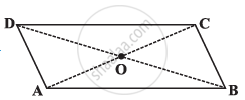Share

# Given a Parallelogram Abcd. Complete Each Statement Along with the Definition Or Property Used Ad = ∠Dcb = - CBSE Class 8 - Mathematics

ConceptKinds of Quadrilaterals Concept of Parallelogram

#### Question

Given a parallelogram ABCD. Complete each statement along with the definition or property used.(2) ∠DCB = …

(3) OC = …

(4) m∠DAB + m∠CDA = …

#### Solution

1) In a parallelogram, opposite sides are equal in length.

2) In a parallelogram, opposite angles are equal in measure.

∠DCB = ∠DAB

3) In a parallelogram, diagonals bisect each other.

Hence, OC = OA

4) In a parallelogram, adjacent angles are supplementary to each other.

Hence, m∠DAB + m∠CDA =180°

Is there an error in this question or solution?

#### APPEARS IN

NCERT Solution for Mathematics Textbook for Class 8 (2018 to Current)